In :
# %load ../../preconfig.py
%matplotlib inline
import matplotlib.pyplot as plt
import seaborn as sns
sns.set(color_codes=True)
plt.rcParams['axes.grid'] = False

#import numpy as np
#import pandas as pd

#import sklearn

#import itertools

import logging
logger = logging.getLogger()


# 24 Single-Source Shortest Paths¶

In a shortest-paths problem,
Given: a weighted, directed graph $G = (V, E)$, with weight function $w : E \to \mathcal{R}$.
path $p = < v_0, v_1, \dotsc, v_k >$, so $$w(p) = \displaystyle \sum_{i=1}^k w(v_{i-1}, v_i)$$.

we define the shortest-path weight $\delta(u, v)$ from $u$ to $v$ by \begin{equation} \delta(u, v) = \begin{cases} \min{ w(p) : u \overset{p}{\to} v } & \text{if $p$ exist}\\ \infty & \text{otherwise} \end{cases} \end{equation}

variants:

• Single-destination shortest-paths problem
• Single-pair shortest-path problem
• All-pairs shortest-path problem

Optimal substructure of a shortest path:
subpath of $p$ is a shortest path between two of its internal nodes if $p$ is a shortest path.

Negative-weight edges: whether a negative weight cycle exists?

Cycles:

• negative-weight cycle: detect and remove
• positive-weight cycle: auto remove
• 0-weight cycle: detect and remove

Representing shortest paths:
a "shortest-paths tree": a rooted tree containing a shortest path from the source $s$ to every vertex that is reachable from $s$.

Relaxation:
modify the node's upper bound if detect a shorter path.

INITIALIZE-SINGLE-SOURCE(G, s)
for each vertex v in G.V
v.d = infty
v.pi = NIL
s.d = 0

RELAX(u, v, w)
if v.d > u.d + w(u, v)
v.d = u.d + w(u, v)
v.pi = u

Properties of shortest paths and relaxtion

• Triangle inequality
• Upper-bound property
• No-path property
• Convergence property
• Path-relaxation property
• Predecessor-subgraph property

### 24.1 The Bellman-Ford algorithm¶

it returns a boolean valued indicating whether or not there is a negative-weight cycle that is reachable from the source.

The algorithm relaxes edges, progressively decreasing an estimate $v.d$ on the weight of a shortest path from the source $s$ to each vertex $v \in V$ until it achieves the actual shortest-path weight $\delta(s, v)$.

The Bellman-Fold algorithm runs in time $O(VE)$.

In :
plt.imshow(plt.imread('./res/bellman_ford.png'))

Out:
<matplotlib.image.AxesImage at 0x117088d68>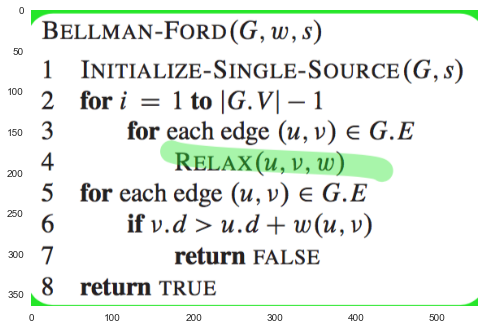In :
plt.imshow(plt.imread('./res/fig24_4.png'))

Out:
<matplotlib.image.AxesImage at 0x117eee518>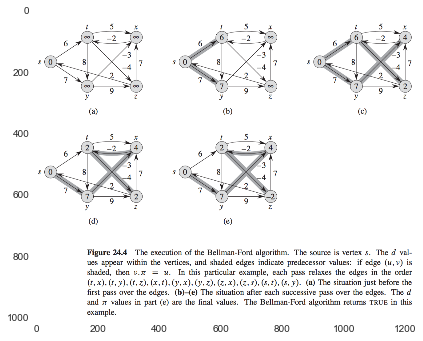### 24.2 Single-source shortest paths in directed acyclic graphs¶

By relaxing the edges of a weighted dag (directed acyclic graph) $G = (V, E)$ according to a topological sort of its vertices, we can compute shortest paths from a single source in $O(V + E)$ time.

In :
plt.imshow(plt.imread('./res/dag.png'))

Out:
<matplotlib.image.AxesImage at 0x11c3c2470>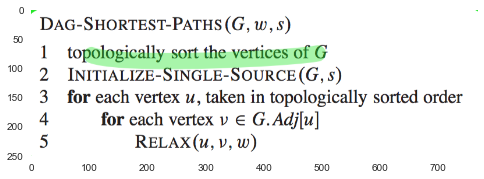In :
plt.imshow(plt.imread('./res/fig24_5.png'))

Out:
<matplotlib.image.AxesImage at 0x11c955748>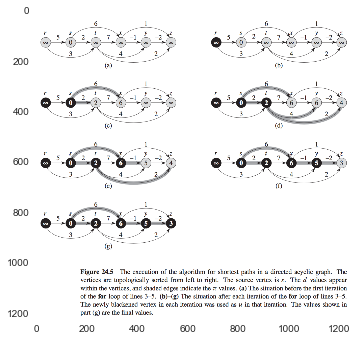interesting application: to determine critical paths in PERT chart analysis.

### 24.3 Dijkstra's algorithm (greedy strategy)¶

weighted, directed graph $G = (V, E)$ and all $w(u, v) \geq 0$.

Dijkstra's algorithm maintains a set $S$ of vertices whose final shortest-path weights from the source $s$ have already been determined.

we use a min-priority queue $Q$ of vertices, keyed by their $d$ values.

In :
plt.imshow(plt.imread('./res/dijkstra.png'))

Out:
<matplotlib.image.AxesImage at 0x121c68c18>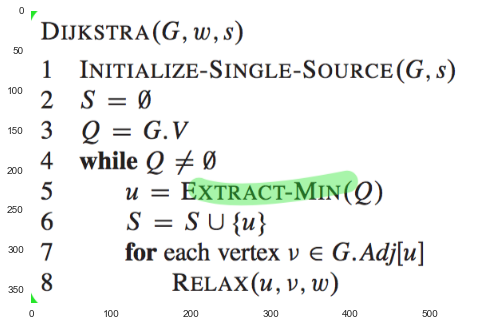In :
plt.imshow(plt.imread('./res/fig24_6.png'))

Out:
<matplotlib.image.AxesImage at 0x12291a6d8>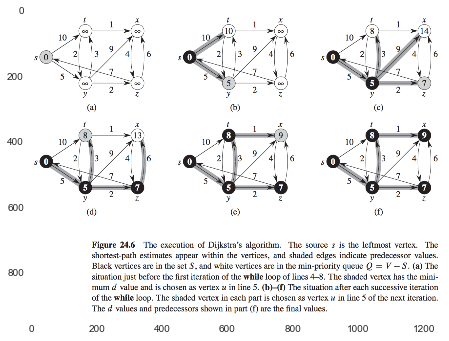### 24.4 Difference constraints and shortest paths¶

#### Systems of difference constraints¶

In a system of difference constraints, each row of the linear-programming matrix $A$ contains one 1 and one -1, and all other entries of $A$ are 0. $\to$ the form $x_j - x_i \leq b_k$.

In :
plt.imshow(plt.imread('./res/inequ.png'))

Out:
<matplotlib.image.AxesImage at 0x123507940>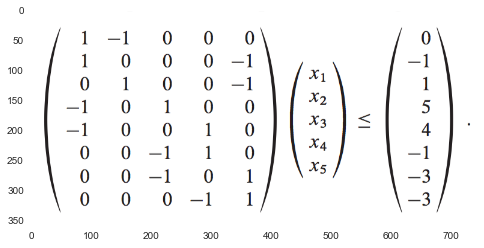#### Constraint graphs¶

In :
plt.imshow(plt.imread('./res/fig24_8.png'))

Out:
<matplotlib.image.AxesImage at 0x1244d3b38>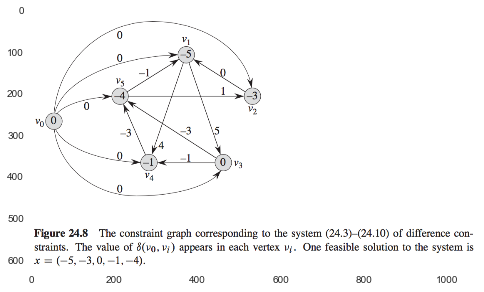### 24.5 Proofs of shortest-paths properties¶

In [ ]: